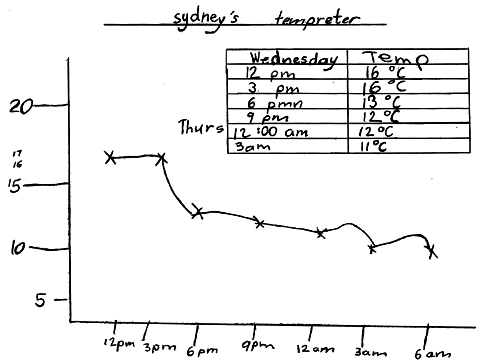Home > Patterns > Misunderstandings > Number lines > Drawing and reading number lines

# Drawing and reading number lines

Students frequently fail to keep the spaces equal when drawing the marks on a number line. It may be necessary to remind students of this very important pattern, especially when labelling the axes of graphs.

Reading off intermediate values on a number line requires students to divide the space between two numbered points into parts (commonly two, five or ten).

For 24-hour or 12-month timelines, the divisions are more likely to be into three, four or six parts.

Sometimes these divisions are marked, but often they are not. Students often find reading off intermediate values quite difficult.

Here is an example from a year 3 classroom.Student's temperature graph.

Note that:

• the marks on both axes are unevenly spaced
• the intermediate values on the vertical axis are in approximately the correct position but have not been located systematically
• the vertical axis would have been more accurate if the student had drawn a number line upwards from 5, marking equal spaces and only labelling the values 10, 15 and 20.

To make tasks like these easier, it is important that students thoroughly understand the structure of the number line from an early age.